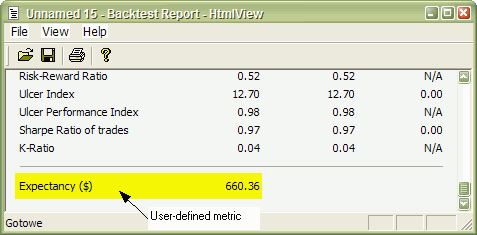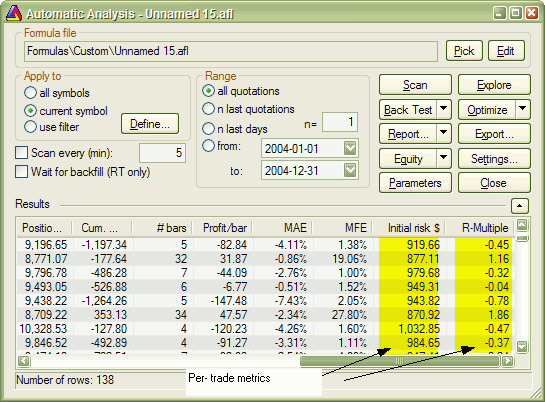## How to add user-defined metrics to backtest/optimization report

One of the new additions in 4.67.x/4.68.x BETA is portfolio backtester programming interface providing full control of 2nd phase of portfolio backtest. This allows multitude of applications including, but not limited to:

• user-defined metrics (appear as an additional column in "summary" backtest result list, in optimization result and as a new row in backtest report "statistics" page, as well as per-trade metrics)
• access to portfolio-level equity when backtest loop is run - allows for example complext position sizing based on portfolio equity
• read/write access on bar-by-bar basis to trading signals generated in 1st backtest phase allows reading ranking array and modifying signal price (for example custom slippage formulas), position size, etc
• access to list of currently open positions, each position can be queried for various properties including profit, MAE/MFE, bars in trade, etc
• three different levels of programming:
• high-level - using Backtest() method and it runs default backtest procedure (as in old versions) - great for adding custom metrics
• mid-level - using PreProcess()/ProcessTradeSignal()/PostProcess() methods - allows to modify signals, query open positions (good for advanced position sizing)

Technical reference of new interface is available here, in this chapter we will just focus on some practical examples.

Example 1

Let's start with the easiest application: in the very first example I will show you how to add user-defined metric to portfolio report and optimization result list.

In the first step we will add Expectancy to backtest and optimization report. There is some discussion about how expectancy should be calculated but the easiest formula for it is:

Expectancy (\$) = %Winners * AvgProfit - %Losers * AvgLoss

or (the other way of calculating the same)

Expectancy (\$) = (TotalProfit - TotalLoss) / NumberOfTrades = NetProfit / NumberOfTrades

Let us start with this simple formulation. With this approach expectancy simply tells us expected profit per trade in dollars. The custom backtest formula that implements this user-defined metric looks as follows:

```/* First we need to enable custom backtest procedure and ** tell AmiBroker to use current formula */ SetCustomBacktestProc(""); /* Now custom-backtest procedure follows */ if( Status("action") == actionPortfolio ) {     bo = GetBacktesterObject();     bo.Backtest(); // run default backtest procedure     st = bo.GetPerformanceStats(0); // get stats for all trades     // Expectancy calculation (the easy way)     // %Win * AvgProfit - %Los * AvgLos     // note that because AvgLos is already negative     // in AmiBroker so we are adding values instead of subtracting them     // we could also use simpler formula NetProfit/NumberOfTrades     // but for the purpose of illustration we are using more complex one :-)     expectancy = st.GetValue("WinnersAvgProfit")*st.GetValue("WinnersPercent")/100 +                 st.GetValue("LosersAvgLoss")*st.GetValue("LosersPercent")/100;     // Here we add custom metric to backtest report     bo.AddCustomMetric( "Expectancy (\$)", expectancy ); } // your trading system here fast = Optimize("fast", 12, 5, 20, 1 ); slow = Optimize("slow", 26, 10, 25, 1 ); Buy=Cross(MACD(fast,slow),Signal(fast,slow)); Sell=Cross(Signal(fast,slow),MACD(fast,slow)); ```

First we need to tell AmiBroker to use custom backtest formula instead of built-in one. We are doing so by calling SetCustomBacktestProc. First parameter defines the path to the custom backtest formula (which can be stored in some external file, independent from actual trading system). If we provide empty string there, we are telling AmiBroker to use current formula (the same which is used for trading system).

In the next line we have "if" statement that enters custom backtest formula if the analysis engine is in actionPortfolio (2nd phase of portfolio backtest) stage. This is important as formula is executed in both scanning phase (when trading signals are generated) and in actual portfolio backtest phase. "if" statement allows us to enter custom backtest procedure part only when analysis engine is in actual backtesting phase.

In the next line we obtain the access to backtester programming interface by calling GetBacktesterObject function. This returns Backtester object that is used to access all functionality of new interface (more details on objects available see: http://www.amibroker.com/docs/ab401.html)

Later we obtain access to built-in metrics by calling GetPerformanceStats method of backtester object. This method returns Statistics object that allows us to access any built-in metric by calling GetValue method.

As a next step we calculate expectancy value from built-in metrics retrieved using GetValue method. For the list of metrics supported by GetValue method please check: http://www.amibroker.com/docs/ab401.html

In the final step we simply add our custom metric to the report by calling AddCustomMetric function of Backtester object. The first parameter is the name of the metric, the second is the value.

After "if"-statement implementing our custom backtest procedure usual trading system rules follow.

Now when you run Backtest and click Report button in Automatic Analysis window you will see your custom metric added at the bottom of statistics page:User-defined metric also appears in the Optimization result list:When you click on the custom metric column, the optimization results will be sorted by your own metric and you will be able to display 3D chart of your user-defined metric plotted against optimization variables.Example 2

Some people point out that this simple method of calculating expectancy works well only with constant position size. Otherwise, with variable position sizing and/or compounding, larger trades weight more than smaller trades and this leads to misleading expectancy values. To address this problem one could calculate expectancy for example as expected profit per \$100 invested. To do calculate such statistic, one needs to iterate through trades, summing up profits per \$100 unit, and dividing this sum by the number of trades. Appropriate formula follows:

```/* First we need to enable custom backtest procedure and ** tell AmiBroker to use current formula */ SetCustomBacktestProc(""); /* Now custom-backtest procedure follows */ if( Status("action") == actionPortfolio ) {     bo = GetBacktesterObject();     bo.Backtest(); // run default backtest procedure    SumProfitPer100Inv = 0;    NumTrades = 0;    // iterate through closed trades first    for( trade = bo.GetFirstTrade(); trade; trade = bo.GetNextTrade() )    {       // here we sum up profit per \$100 invested        SumProfitPer100Inv = SumProfitPer100Inv + trade.GetPercentProfit();        NumTrades++;    }    // iterate through eventually still open positions    for( trade = bo.GetFirstOpenPos(); trade; trade = bo.GetNextOpenPos() )    {        SumProfitPer100Inv = SumProfitPer100Inv + trade.GetPercentProfit();        NumTrades++;    }    expectancy2 = SumProfitPer100Inv / NumTrades;     bo.AddCustomMetric( "Expectancy (per \$100 inv.)", expectancy2 ); } // your trading system here fast = Optimize("fast", 12, 5, 20, 1 ); slow = Optimize("slow", 26, 10, 25, 1 ); Buy=Cross(MACD(fast,slow),Signal(fast,slow)); Sell=Cross(Signal(fast,slow),MACD(fast,slow)); ```

The only difference between this and previous formula is that we do not use built-in metrics to calculate our own expectancy figure. Instead we sum up all percentage profits of each trade (which are equivalent to dollar profits from \$100 unit investment) and at the end divide the sum by the number of trades. Summing up is done inside the "for" loop. GetFirstTrade/GetNextTrade function pair of the backtester object allows us to step through the list of closed trades. We use two loops (second loop uses GetFirstOpenPos/GetNexOpenPos) because there may be some open positions left at the end of the backtest. If we wanted to include only closed trades then we could remove second "for" loop.

After running this code we find out that expectancy calculated this way even adjusted to initial equity (by multiplying by factor InitialEquity/\$100) is smaller than expectancy calculated in the first example. This shows that "easy" method of expectancy calculation (from example 1) may lead to overly optimistic results.

Example 3

Some Van Tharp followers prefer yet slightly differnt "twist" of expectancy measure. They express expectancy in terms of expected profit per "unit of risk". The profit is then expressed in terms of R-multiples, where 1R is defined as the amount risked per trade. The amount risked is the maximum amount of money you can lose, and most often it is set by the amount of maximum loss stop (or trailing stop). According to Tharp, the easiest way to calculate expectancy is simply to add up all your R-multiples and net them out by subtracting the negative R-multiples from the positive ones, then divide by the no. of trades. This gives you your expectancy per trade.

This is very similar to approach presented in example 2, but for the calculations we do not use the value of the trade but rather risk per trade. The risk depends on the stop we use in our trading system. For simplicity in this example we have used 10% max. loss stop. In this example we also add per-trade metrics for better illustration of how R-multiples are calculated. Per-trade metrics appear in each row of the trade list in the backtest results.The formula that implements this kind of expectancy measure follows:

```/* First we need to enable custom backtest procedure and ** tell AmiBroker to use current formula */ SetCustomBacktestProc(""); MaxLossPercentStop = 10; // 10% max. loss stop /* Now custom-backtest procedure follows */ if( Status("action") == actionPortfolio ) {     bo = GetBacktesterObject();     bo.Backtest(1); // run default backtest procedure    SumProfitPerRisk = 0;    NumTrades = 0;    // iterate through closed trades first    for( trade = bo.GetFirstTrade(); trade; trade = bo.GetNextTrade() )    {       // risk is calculated as the maximum value we can loose per trade       // in this example we are using  max. loss stop       // it means we can not lose more than (MaxLoss%) of invested amount       // hence ris        Risk = ( MaxLossPercentStop / 100 ) * trade.GetEntryValue();        RMultiple = trade.GetProfit()/Risk;        trade.AddCustomMetric("Initial risk \$", Risk  );        trade.AddCustomMetric("R-Multiple", RMultiple  );        SumProfitPerRisk = SumProfitPerRisk + RMultiple;        NumTrades++;    }     expectancy3 = SumProfitPerRisk / NumTrades;     bo.AddCustomMetric( "Expectancy (per risk)", expectancy3 );     bo.ListTrades(); } // your trading system here ApplyStop( stopTypeLoss, stopModePercent, MaxLossPercentStop ); fast = Optimize("fast", 12, 5, 20, 1 ); slow = Optimize("slow", 26, 10, 25, 1 ); Buy=Cross(MACD(fast,slow),Signal(fast,slow)); Sell=Cross(Signal(fast,slow),MACD(fast,slow)); ```